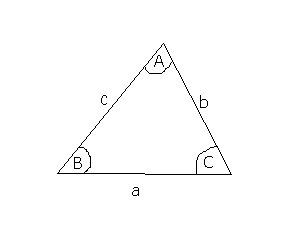## Sine and Cosine Rule

This section looks at the Sine Law and Cosine Law.

The Sine Rule

The Law of Sines (sine rule) is an important rule relating the sides and angles of any triangle (it doesn't have to be right-angled!):

If a, b and c are the lengths of the sides opposite the angles A, B and C in a triangle, then:

a   =

sinA    sinB  sinCIf you wanted to find an angle, you can write this as:

sinAsinB  = sinC

a         b           c

This video shows you how to use the Sine rule

The Cosine Rule

This also works in any triangle:

c² = a² + b² - 2abcosC
which can also be written as:
a² = b² + c² - 2bccosA

This video show you how to use the Cosine rule

The area of a triangle

The area of any triangle is ½ absinC (using the above notation).
This formula is useful if you don't know the height of a triangle (since you need to know the height for ½ base × height).